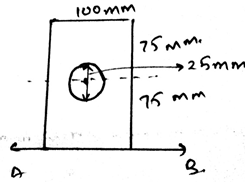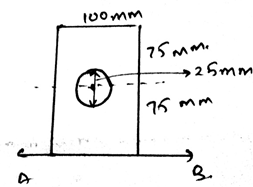Courses

# Test: Moment Of Inertia For Composite Areas

## 15 Questions MCQ Test Engineering Mechanics | Test: Moment Of Inertia For Composite Areas

Description
This mock test of Test: Moment Of Inertia For Composite Areas for Mechanical Engineering helps you for every Mechanical Engineering entrance exam. This contains 15 Multiple Choice Questions for Mechanical Engineering Test: Moment Of Inertia For Composite Areas (mcq) to study with solutions a complete question bank. The solved questions answers in this Test: Moment Of Inertia For Composite Areas quiz give you a good mix of easy questions and tough questions. Mechanical Engineering students definitely take this Test: Moment Of Inertia For Composite Areas exercise for a better result in the exam. You can find other Test: Moment Of Inertia For Composite Areas extra questions, long questions & short questions for Mechanical Engineering on EduRev as well by searching above.
QUESTION: 1

### A ______________ body consists of a series of connected “simpler” shaped bodies.

Solution:

A composite body consists of a series of connected simpler shaped bodies. That is the body is having two material as it’s constitutes. The main thing is that the single material is having the properties of two different materials.

QUESTION: 2

### Composite materials are of no use for the any engineers.

Solution:

The composite materials are of much importance to the chemical engineer. This means that the composite materials are variedly used in the chemical industries. It can be used in the bridges made for the pipelines, etc.

QUESTION: 3

### The composite materials are lighter than their constituent material.

Solution:

Yes the composite materials are lighter than the constituent material. This means that the density of the former is lighter than the latter. Thus imparting many qualities to the materials. Thus the material can be used variedly. This means that the moment of inertia of the structure is low.

QUESTION: 4

Determine the moment of area of the area below about x-axis.Solution:

The product of the centroid of the section to its mass to the total mass of the body is the centre of mass. Thus the answer. The ratio is generally used to locate the coordinate of the centroid or the centre of mass.

QUESTION: 5

Composite materials can be of __________ shapes for the determination of the moment of composite areas.

Solution:

The composite materials can be of any shape. This means that the shape of the material can be varied. Thus any shape can be imparted to the composite materials and then be used in the industries variedly.

QUESTION: 6

The total of all the masses of small particles adds up to give the total body mass of the composite body. This mass lies along with gravity gives a force vector which is being passed by ________

Solution:

The total body mass along with the product of gravity always passes through the centre of gravity. All the forces are parallel is the right answer. Thus the total sum of all these small weights add up to the total weight of the body. Which passes through the centre of gravity.

QUESTION: 7

Determine the moment of area of the area below its centroid axis.Solution:

The product of the centroid of the section to its mass to the total mass of the body is the centre of mass. Thus the answer. The ratio is generally used to locate the coordinate of the centroid or the centre of mass.

QUESTION: 8

One of the use of the centre of mass or centroid of the composite bodies is as in the simplification of the loading system the net force acts at the ___________ of the loading body.

Solution:

In the simplification of the loading system the net force acts at the centroid of the composite body. That is if the loading system is in the form of the triangle then at the distance 2 by 3 of the base the net force of the loading will act. And the load will be half the area of the loading.

QUESTION: 9

If any external force also is applied on the distributed loading on which the moment of inertia is to be determined on the composite body then?

Solution:

The external forces are treated differently. They are not added by the force of the distributed loading. That is the force not only acts at the centroid always. It can be shifted also. Depending on the external forces. Thus the use of centroid or centre of mass.

QUESTION: 10

Two of the things of the composite materials are to be known so that their moment of inertia can be varied. Which of the following is one of them?

Solution:

The centre of the gravity’s location and the weight of the composite body is needed to be known. This means that if both of the quantities of the composite body is known then the moment of inertia of the body can be altered. Thus both are very important things to be known about the composite bodies.

QUESTION: 11

The number of particle does affect the moment of inertia. A composite body is consisted of __________ number of particles.

Solution:

The various bodies are composed of infinite number of particles. They are very small in size. They can’t be seen by our naked eyes. Thus the number of particles is not thousands nor hundreds. But it is uncountable.

QUESTION: 12

We use sometimes the measures to know the direction of moment of inertia. It is done by right handed coordinate system. Which is right about it for the composite bodies (consider the mentioned axis to be positive)?

Solution:

As right handed coordinate system means that you are curling your fingers from positive x-axis towards y-axis and the thumb which is projected is pointed to the positive z-axis. Thus visualizing the same and knowing the basic members of axis will not create much problem.

QUESTION: 13

We often use the centre of mass of the composite area to calculate the moment of inertia. The centre of mass for these composite body is the ratio of ________ to _________

Solution:

The product of the centroid of the section to its mass to the total mass of the body is the centre of mass. Thus the answer. The ratio is generally used to locate the coordinate of the centroid or the centre of mass.

QUESTION: 14

What is not the condition for the equilibrium in three dimensional system of axis for the composite bodies if we are determining the moment of inertia for them?

Solution:

For the equilibrium in the three dimensional system of axis we have all the conditions true as, ∑Fx=0, ∑Fy=0 and ∑Fz=0. Also we have the summation of the forces equal to zero. Which is not a non-zero value.

QUESTION: 15

We sometimes use the moment caused by the force so as to determine the moment of inertia of the body. The moment is the cross product of which two vectors?

Solution:

The cross product needs to take in the proper sequence. If not taken then the answer is just the opposite of the true answer. That’s why, the answer is not the Force and Radius vectors, but the Radius and Force vectors. Because the moment has its direction, as many of the cross products have, and thus precaution needs to be taken.